## 高速列车车体长度对气动噪声影响的数值研究 1)

* 中国科学院力学研究所流固耦合系统力学重点实验室, 北京 100190

† 北京理工大学宇航学院力学系, 北京 100081

## INFLUENCE OF THE LENGTH OF HIGH-SPEED TRAIN ON THE FAR-FIELD AEROACOUSTICS CHARACTERISTICS 1)

Mo Huangrui*, An Yi*, Liu Qingquan,,2)

* Institute of Mechanics, Chinese Academy of Sciences, Beijing 100190, China

† School of Aerospace Engineering, Beijing Institute of Technology, Beijing 100081

 基金资助: 1) 中国科学院知识创新工程方向性资助项目.  KJCX2-EW-L02-1Abstract

The estimation of the aeroacoustic characteristics of the high-speed train is of great importance in train design. Due to the extreme slender shape of the full marshaling of the train (generally 8 or 16 cars), the calculation of the far filed acoustics involves massive consumption of the CPU time which even makes the optimization unpractical. This paper numerically studies the influence of train length on its aeroacoustics characteristics by considering the acoustic contribution car by car. The nonlinear acoustic solver (NLAS) is used to calculate the acoustic source at the acoustic surface, and the FW-H analogy method is used to integrate on the acoustic surface to obtain the far-field results. Three different marshaling of the trains, i.e. 3 cars, 4 cars, and 6 cars, are studied and compared. The far-field acoustic level and frequency profile along the train is obtained. The results show that for different middle cars, the far-field acoustic profile along the length is very similar in both quantity and shape, except the offset in position. For the cars in the same position of different marshaling, both the sound pressure level distribution and the frequency profile are also very close to each other. Thus the key aeroacoustics characteristics of a long marshaling train could be estimated with a much smaller marshaling such as 3 cars. With the superposition of the acoustic surface data from short marshaling simulation, the aeroacoustics characteristics of long marshaling could be obtained. The comparison of the superposed results and the results calculated directly from long marshaling simulation is close enough for engineering use. This demonstrates that the proposed novel approach for estimating aeroacoustics of long marshaling could not only reduce the computational cost significantly but also be as accurate as direct simulation. This paper might provide a handy tool for engineering practice in this region.

Keywords： high-speed train ; aerodynamic noise ; NLAS ; FW-H acoustic analogy ; long marshalling

Mo Huangrui, An Yi, Liu Qingquan. INFLUENCE OF THE LENGTH OF HIGH-SPEED TRAIN ON THE FAR-FIELD AEROACOUSTICS CHARACTERISTICS 1). Chinese Journal of Theoretical and Applied Mechanics[J], 2019, 51(5): 1310-1320 DOI:10.6052/0459-1879-19-079

## 1 数值模拟方法

$$\dfrac{\partial { q}'}{\partial t} + \dfrac{\partial { F}'_i }{\partial x_i } - \dfrac{\partial (F_i^v {)}'}{\partial x_i } = - \dfrac{\partial \bar { q}}{\partial t} - \dfrac{\partial \bar { F}_i }{\partial x_i } + \dfrac{\partial \bar { F}_i^v }{\partial x_i }$$

$$\bar { q} = \left[ {{\begin{array}{*{20}c} \bar {\rho } \\ {\bar {\rho }\bar {u}_j } \\ \bar {e} \\ \end{array} }} \right],\quad F_i = \left[ {{\begin{array}{*{20}c} {\bar {\rho }\bar {u}_i } \\ {\bar {\rho }\bar {u}_i \bar {u}_j + \bar {p}\delta _{ij} } \\ {\bar {u}_i (\bar {e} + \bar {p}\_} \\ \end{array} }} \right] \\ F_i^v = \left[ {{\begin{array}{*{20}c} 0 \\ {\bar {\tau }_{ij} } \\ { - \bar {\theta }_i + \bar {u}_k \bar {\tau }_{ki} } \\ \end{array} }} \right] \\ { q}' = \left[ {{\begin{array}{*{20}c} \rho \\ {\bar {\rho }{u}'_j + {\rho }'\bar {u}_j + {\rho }'{u}'_j } \\ {e}' \\ \end{array} }} \right] \\ ( F_i^v {)}' = \left[ {{\begin{array}{*{20}c} 0 \\ {{\tau }'_{ij} } \\ { - {\theta }'_i + {u}'_k \bar {\tau }_{ki} + \bar {u}_k {\tau }'_{ki} } \\ \end{array} }} \right] \\ { F}'_i = \left[ {{\begin{array}{*{20}c} {\bar {\rho }{u}'_i + {\rho }'\bar {u}_i } \\ {{\rho }'\bar {u}_i \bar {u}_j + \bar {\rho }\bar {u}_i {u}'_j + \bar {\rho }{u}'_i \bar {u}_j + {p}'\delta _{ij} } \\ {{u}'_i (\bar {e} + \bar {p}) + \bar {u}_i ({e}' + {p}')} \\ \end{array} }} \right]+ \\ \qquad \left[ {{\begin{array}{*{20}c} {{\rho }'{u}'_i } \\ {\bar {\rho }{u}'_i {u}'_j + {\rho }'{u}'_i \bar {u}_j + {\rho }'\bar {u}_i {u}'_j + {\rho }'{u}'_i {u}'_j } \\ {{u}'_i ({e}' + {p}')} \\ \end{array} }} \right]$$

$$R_i = \left[ {{\begin{array}{*{20}c} 0 \\ {\overline {\bar {\rho }{u}'_i {u}'_j } } \\ {\overline {c_p \rho {T}'{u}'_i } + \overline {\bar {\rho }{u}'_i {u}'_k } \bar {u}_k + \textstyle{1 \over 2}\overline {\bar {\rho }{u}'_k {u}'_k {u}'_i } + \overline {{u}'_k \tau _{ki} } } \\ \end{array} }} \right]$$

$$4\pi {p}'({ x},t) = \\ \qquad \iint {\left[ {\dfrac{\dot {Q}_j \hat {n}_j }{r(1 - M_r )^2} + \dfrac{Q_j \hat {n}_j (r\dot {M}_r + c_\infty (M_r - M^2))}{r^2\vert 1 - M_r \vert ^3}} \right]} _{\rm ret} {\rm d}s + \\ \qquad \iint {\left[ {\dfrac{\dot {L}_j \hat {r}_j }{c_\infty r(1 - M_r )^2} + \dfrac{L_j \hat {r}_j - LM}{r^2(1 - M_r )^2}} \right]} _{\rm ret} {\rm d}s+ \\ \qquad \iint {\left[ {\dfrac{L_j \hat {r}_j (r\dot {M}{ }_k\hat {r}{ }_k + c_\infty (M{ }_k\hat {r}{ }_k - M^2))}{c_\infty r^2\vert 1 - M_r \vert ^3}} \right]} _{\rm ret} {\rm d}s + {p}'_Q$$

## 2 计算列车模型与设置

### 图1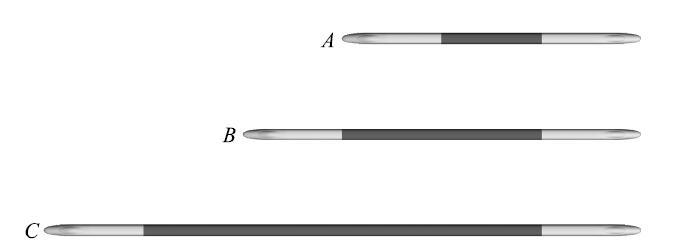Fig. 1   Simplified models of three trains with different lengths

### 图2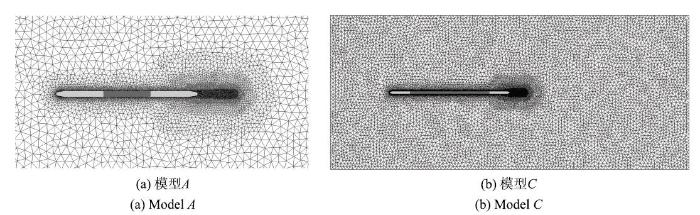Fig. 2   Computational mesh grid for different trains

## 3 计算结果

### 图3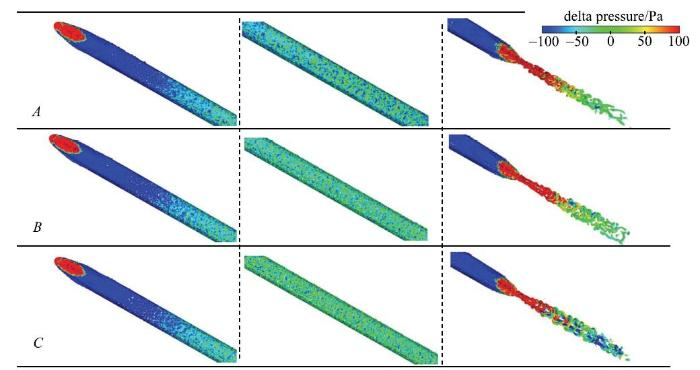Fig. 3   Isosurface of Q criterion of different trains colored by $\Delta p =p-p_{\infty , }Q$= ($U_{\infty }$/$H)^{2}$

### 图4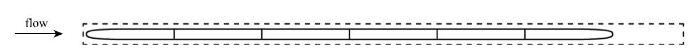Fig. 4   Location of the acoustic surfaces for Model $C$

### 图5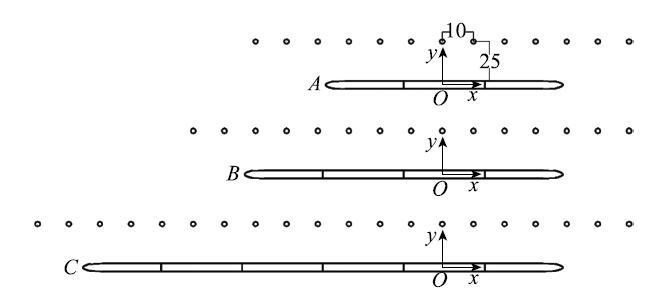Fig. 5   Location of sound pressure probes along with the trains

### 图6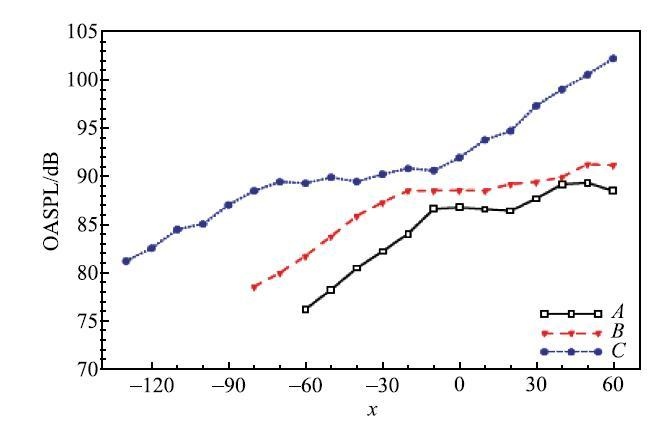Fig. 6   Overall sound pressure level profile along the trains of different lengths

### 图7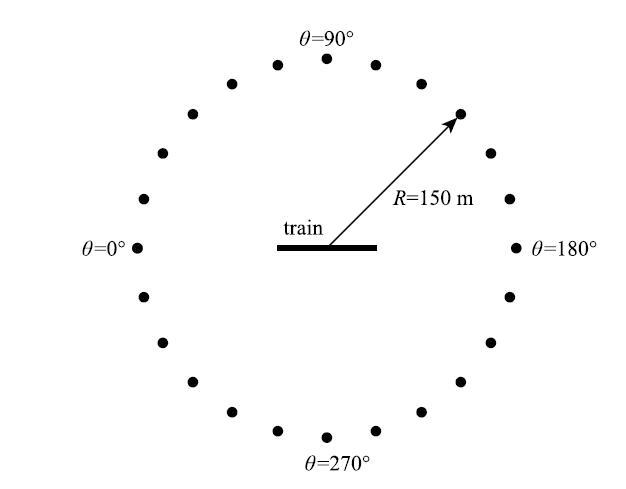Fig. 7   Location of sound pressure probes around the trains

### 图8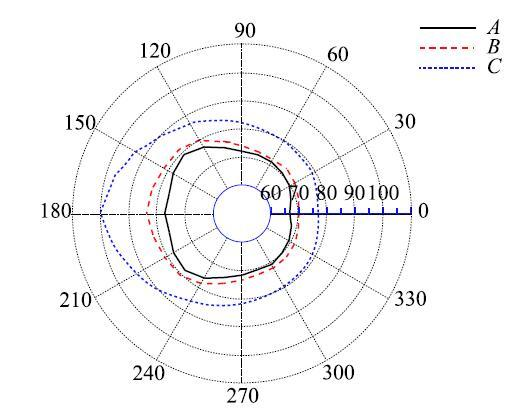Fig. 8   OASPL around the trains with different lengths

## 4 分析和讨论

### 图9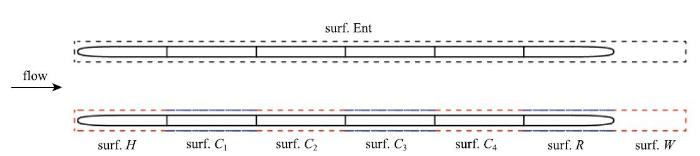Fig. 9   Acoustic surface setup for model $C$

### 图10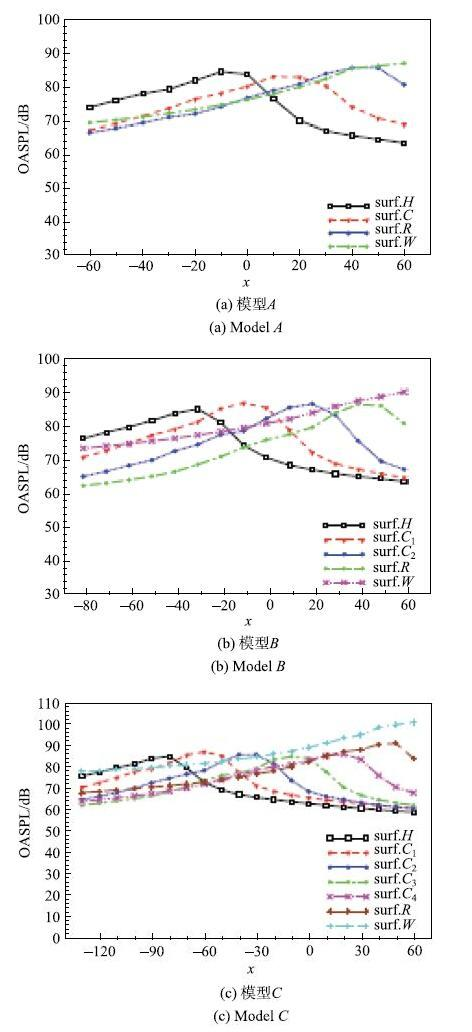Fig. 10   OASPL profile along the train of different lengths

### 图11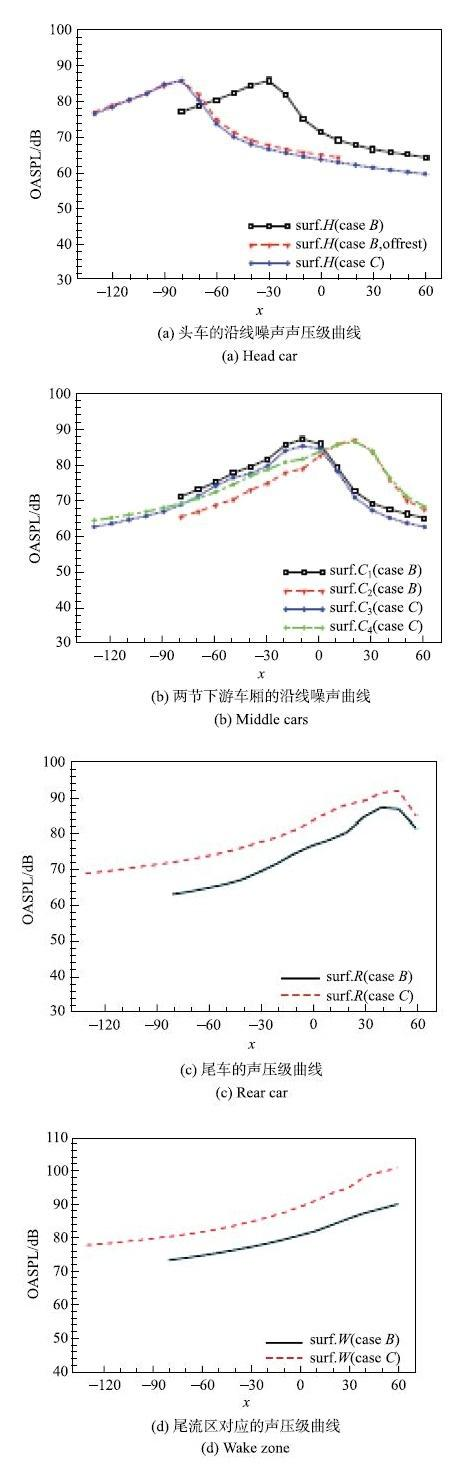Fig. 11   OASPL profile comparison car by car for model $B${\&} $C$

### 图12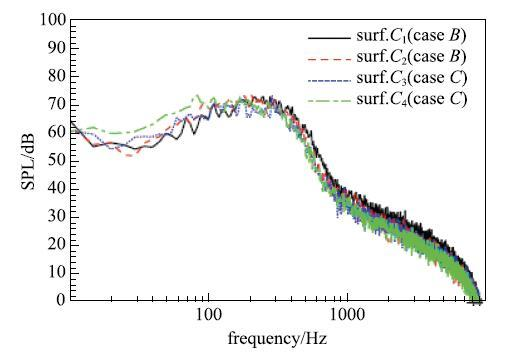Fig. 12   Spectrum of SPL for middle cars at the given location

### 图13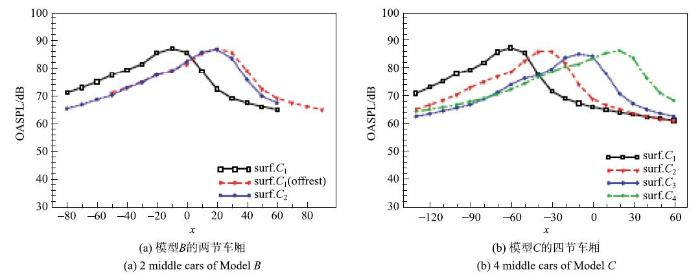Fig. 13   OASPL profile along the train for different cars of model

### 图14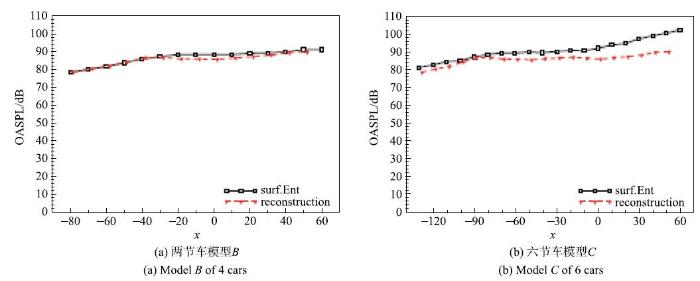Fig. 14   Reconstructed OASPL profile along the train for model $B$& $C$using data from the simulation of model $A$

## 5 结 论

(1) 车体长度的增大基本不会改变列车远场噪声的分布规律,但将显著增加远场噪声的声压级,说明用短编组模型计算得到的噪声明显低估了长编组列车的气动噪声.

(2) 同一列车模型的各节车厢具有相似的沿线噪声,其噪声曲线在量值上十分接近,只是主峰位置会随着车厢空间位置的不同而相应地发生偏移,且偏移距离与相应车厢间的空间距离一致.当得到模型的某单节车厢的远场噪声曲线时,则其他车厢的远场噪声特性可由已知车厢的远场噪声曲线通过合适的空间偏移得到.

(3) 不同长度编组列车对应部位之间的远场噪声特性具有较强的关联性,它们的远场噪声具有接近的总声压级和相吻合的噪声频谱,为基于短编组列车远场噪声结果重构长编组列车的远场噪声提供了可能性.

(4) 基于三节车编组列车噪声的数值计算结果,重构得到的四节车与六节车的沿线噪声, 与直接数值计算的结果吻合较好,由此说明, 基于短编组列车噪声结果, 通过重构和叠加,可以实现对更长编组列车

## 参考文献 原文顺序 文献年度倒序 文中引用次数倒序 被引期刊影响因子

Talotte C .

Aerodynamic noise: A critical survey

Journal of Sound and Vibration, 2000,231(3):549-562

Mellet C, Létourneaux F, Poisson F , et al.

High speed train noise emission: Latest investigation of the aerodynamic/rolling noise contribution

Journal of sound and vibration, 2006,293(3):535-546

( Yang Guowei, Wei Yujie, Zhao Guilin , et al.

Research progress on the mechanics of high speed rails

( Sun Zhenxu, Yao Yongfang, Yang Yan , et al.

Overview of the research progress on aerodynamic noise of high speed trains in China

Acta Aerodynamica Sinica, 2018,36(3):29-41(in Chinese))

( Jin Yongrong, Chen Xiaoli .

Overview of research on noise source distribution and active noise reduction of Shinkansen Trains in Japan

Modern Informationn Technology, 2019,3(3):176-177, 179(in Chinese))

CIT500车外噪声源频谱分解模型的试验研究

( Tan Xiaoming, Yang Zhigang, Wu Xiaolong , et al.

Experimental study on frequency spectrum component model of noise source outside CIT500 train

Journal of the China Railway Society, 2017,39(7):32-37(in Chinese))

Yokoshima S, Morihara T, Sato T , et al.

Combined effects of high-speed railway noise and ground vibrations on annoyance

International Journal of Environmental Research & Public Health, 2017,14(8):845

Kitagawa T, Nagakura K .

Aerodynamic noise generated by shinkansen cars

Journal of Sound and Vibration, 2000,231(3):913-924

Suzuki M, Ikeda M, Yoshida K .

Study on numerical optimization of cross-sectional panhead shape for high-speed train

Journal of Mechanical Systems for Transportation and Logistics, 2008,1:100-110

( Gao Yang, Li Qiliang, Chen Yu , et al.

Prediction of near field and far field noise for high-speed train head shape

Journal of Tongji University(Natural Science), 2019,47(1):124-129(in Chinese))

( Liu Xiaori, Luo Jiangze, Zhao Zhe , et al.

Numerical analysis of influence of head length on aerodynamic and sound field characteristics of g-series high-speed train and research on noise reduction

China Railway Science, 2018,39(5):88-96(in Chinese))

( Liu Guoqing, Du Jian, Liu Jiali , et al.

Investigation on the influence of inter-car windshield types on the aerodynamic noise of high speed trains

Noise and Vibration Control, 2018,38(2):87-90(in Chinese))

Zhu JY, Hu ZW, Thompson DJ .

The effect of a moving ground on the flow and aerodynamic noise behaviour of a simplified high-speed train bogie

International Journal of Rail Transportation, 2017,5(2):110-125

Zhang YD, Zhang JY, Tian LI , et al.

Investigation of the aeroacoustic behavior and aerodynamic noise of a high-speed train pantograph

Science China Technological Sciences, 2017,60(4):75-89

Sun X, Han X .

Numerical modeling and investigation on aerodynamic noise characteristics of pantographs in high-speed trains

Complexity, 2018(6):1-12

( Liu Jiali, Yu Mengge, Tian Aiqin , et al.

Study on the aerodynamic noise characteristics of the pantograph of the high-speed train

Journal of Mechanical Engineering, 2018,54(4):231-237(in Chinese))

( Dong Jilei .

Numerical simulation analysis of aerodynamic noise for pantographs of high-speed trains

Noise and Vibration Control, 2018,38(s1):46-50(in Chinese))

( Liu Jiali, Zhang Jiye, Zhang Weihua .

Numerical analysis on aerodynamics noise of the high-speed train head

Journal of the China Railway Society, 2011,33(9):19-26(in Chinese))

( Xiao Yougang, Kang Zhi-cheng .

Numerical prediction of aerodynamics noise radiated from high-speed train head surface. Journal of

Central South University. 2008,39(6):1267-1272(in Chinese))

CRH3型高速列车气动噪声数值模拟研究

( Sun Zhenxu, Song Jingjing, An Yiran ,

Numerical simulation of aerodynamics noise generated by CRH3 high-speed train

Acta Scientiarum Naturalium Universitatis Pekinensis, 2012,48(5):701-711(in Chinese))

Yu HH, Li JC, Zhang HQ .

On aerodynamic noises radiated by the pantograph system of high-speed trains

Acta Mechanica Sinica, 2013,29(3):399-410

( An Yi, Mo Huangrui, Liu Qingquan .

Study on the influence of the nose slenderness ratio of high-speed train on the aerodynamic noise

Chinese Journal of Theoretical and Applied Mechanics, 2017,49(5):985-996(in Chinese))

( Yuan Lei, Li Renxian ,

Aerodynamics noise of high-speed train and its impact

Mechanical Engineering & Automaytion, 2013,180(5):31-35(in Chinese))

Yang Y, Yang GW .

A numerical study on aerodynamics noise sources of high-speed train

Proceedings of the 1st IWHIR, 2012(2):107-116

( Zhang Jun, Huang Yanyi, Zhao Wenzhong .

Research on numerical simulation of aerodynamics noise for high-speed train

Journal of Dalian Jiaotong University, 2012,33(4):1-5(in Chinese))

( Zhang Jun, Sun Bangcheng, Guo Tao , et al.

Research on aerodynamics noise radiated from whole body surface of high-speed train and its distribution

Journal of the China Railway Society, 2015,37(2):10-17(in Chinese))

( Yu Peixun, Yang Hai, Pan Kai , et al.

A search algorithm coupling parallel strategy and distance decreasing method applied to CAA

Chinese Journal of Computational Mechanics, 2018,35(2):255-260(in Chinese))

Pascioni KA, Cattafesta LN . An aeroacoustic study of a leading-edge slat: Beamforming and far field estimation using near field quantities. Journal of Sound & Vibration}, 2018,429:224-244

Zhang Y, Zhang J, Tian Li . Contribution analysis of aerodynamic noise of high-speed train. Journal of Traffic & Transportation Engineering, 2017(4):78-88

Batten P, Ribaldone E, Casella M , et al. Towards a generalized non-linear acoustics solver. AIAA-2004-3001,Proceeding of 10$^{\rm th}$AIAA/CEAS Aeroacoustics Conference,2004, May 10-12, Manchester, UK

Batten P, Goldberg U , Chakravarthy S. Reconstructed sub-grid methods for acoustics predictions at all Reynolds numbers. AIAA Paper 2002- 2511, 2002

Ffowcs Williams JE, Hawkings DL .

Sound generation by turbulence and surfaces in arbitrary motion. Philosophical Transactions of the Royal Society of London,

Series A, 1969,264:321-342

Farassat F .

Discontinuities in aerodynamics and aeroacoustics: The concept and applications of generalized derivatives

Journal of Sound and Vibration, 1977,55(2):165-193

Powell A .

Theory of vortex sound

The Journal of the Acoustical Society of America, 2005,36(1):177-195

/

 〈〉